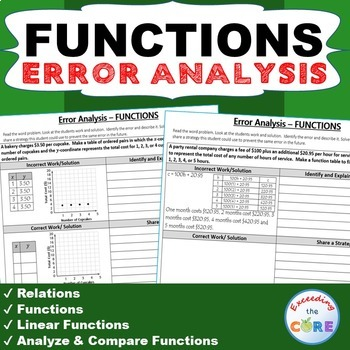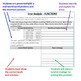# FUNCTIONS Word Problems - Error Analysis (Find the Error)Subject
Resource Type
File Type

PDF

(1 MB|14 pages)
Standards
Also included in:
1. FUNCTIONS BUNDLE - Task Cards, Error Analysis, Graphic Organizers, Maze, Riddle, Coloring Activity This BUNDLE includes 40 Task Cards, 10 error analysis activities and 10 problem solving graphic organizers, 1 maze, 1 riddle, 1 coloring activity (over 90 skills practice and real-world word problems
\$11.00
\$8.80
Save \$2.20
2. Great for online learning and distance learning!Have your students apply their understanding of math concepts with these 8th Grade ERROR ANALYSIS activities. This BUNDLE resource includes 70 questions (7 sets/10 questions per set) of Common Core WORD PROBLEMS that are solved incorrectly. Students
\$21.00
\$16.80
Save \$4.20
3. - Great for online learning and distance learning!This middle school ERROR ANALYSIS BUNDLE includes 230 real-world word problems (23 sets) that are solved incorrectly for grades 6-8. Students have to IDENTIFY THE ERROR, provide the CORRECT SOLUTION and share a helpful STRATEGY for solving the proble
\$69.00
\$48.30
Save \$20.70
• Product Description
• Standards
Have your students apply their understanding of FUNCTIONS with these ERROR ANALYSIS activities.

Benefits of Math Error Analysis:
Giving students opportunities to identify and correct errors in presented solutions allows them to show their understanding of the mathematical concepts you have taught.

Whats Included:
This resource includes 10 real-world FUNCTIONS word problems that are solved incorrectly. Students have to IDENTIFY THE ERROR, provide the CORRECT SOLUTION and share a helpful STRATEGY for solving the problem. An ANSWER KEY has also been provided with examples of possible answers. Be sure to download the sample for a full overview of what you get.

How to Use:
When I present this activity to my students, I tell them that these are errors that students from my other class made and I need their help to correct them. Students LOVE correcting other students mistakes. Students can use these activities with a partner, as a warm-up , as classwork, homework, in math centers or group work.

Be sure to download the sample for a full overview of what you get.

Topics Covered:
✔ Relations
✔ Functions
✔ Linear Functions
✔ Analyze & Compare Functions

Common Core Alignment :
* 8.F.1 Understand that a function is a rule that assigns to each input exactly one output. The graph of a function is the set of ordered pairs consisting of an input and the corresponding output.
* 8.F.2 Compare properties of two functions each represented in a different way (algebraically, graphically, numerically in tables, or by verbal descriptions)
* 8.F.3 Interpret the equation y = mx + b as defining a linear function, whose graph is a straight line; give examples of functions that are not linear.
* 8.F.4 Construct a function to model a linear relationship between two quantities. Determine the rate of change and initial value of the function from a description of a relationship or from two (x, y) values, including reading these from a table or from a graph. Interpret the rate of change and initial value of a linear function in terms of the situation it models, and in terms of its graph or a table of values.
* 8.F.5 Describe qualitatively the functional relationship between two quantities by analyzing a graph (e.g., where the function is increasing or decreasing, linear or nonlinear).
* MP3 - Construct viable arguments and critique the reasoning of others.

BUNDLE UP & SAVE \$\$ with my Functions UNIT RESOURCE BUNDLE

Get it all in my 8th Grade Common Core MEGA-BUNDLE!

More FUNCTIONS resources:
* Functions ERROR ANALYSIS
* Functions PROBLEM SOLVING GRAPHIC ORGANIZER
* Functions MAZE, RIDDLE, COLORING PAGE

* 8th Grade ERROR ANALYSIS BUNDLE
* 8th Grade Winter MATH PACKET
* 8th Grade WORD PROBLEMS WITH GRAPHIC ORGANIZERS BUNDLE

If you would like to get updates on NEW and CURRENT resources...
FOLLOW the Exceeding the Core BLOG!
FOLLOW me on Teachers Pay Teachers!

© Exceeding the CORE. All rights reserved. Please note - this resource is for use by one teacher only. Additional teachers must purchase their own license. Copying, editing, selling, redistributing, or posting any part of this product on the internet is strictly forbidden. Violations are subject to the penalties of the Digital Millennium Copyright Act.
Construct viable arguments and critique the reasoning of others. Mathematically proficient students understand and use stated assumptions, definitions, and previously established results in constructing arguments. They make conjectures and build a logical progression of statements to explore the truth of their conjectures. They are able to analyze situations by breaking them into cases, and can recognize and use counterexamples. They justify their conclusions, communicate them to others, and respond to the arguments of others. They reason inductively about data, making plausible arguments that take into account the context from which the data arose. Mathematically proficient students are also able to compare the effectiveness of two plausible arguments, distinguish correct logic or reasoning from that which is flawed, and-if there is a flaw in an argument-explain what it is. Elementary students can construct arguments using concrete referents such as objects, drawings, diagrams, and actions. Such arguments can make sense and be correct, even though they are not generalized or made formal until later grades. Later, students learn to determine domains to which an argument applies. Students at all grades can listen or read the arguments of others, decide whether they make sense, and ask useful questions to clarify or improve the arguments.
Describe qualitatively the functional relationship between two quantities by analyzing a graph (e.g., where the function is increasing or decreasing, linear or nonlinear). Sketch a graph that exhibits the qualitative features of a function that has been described verbally.
Construct a function to model a linear relationship between two quantities. Determine the rate of change and initial value of the function from a description of a relationship or from two (𝘹, 𝘺) values, including reading these from a table or from a graph. Interpret the rate of change and initial value of a linear function in terms of the situation it models, and in terms of its graph or a table of values.
Interpret the equation 𝘺 = 𝘮𝘹 + 𝘣 as defining a linear function, whose graph is a straight line; give examples of functions that are not linear. For example, the function 𝘈 = 𝑠² giving the area of a square as a function of its side length is not linear because its graph contains the points (1,1), (2,4) and (3,9), which are not on a straight line.
Compare properties of two functions each represented in a different way (algebraically, graphically, numerically in tables, or by verbal descriptions). For example, given a linear function represented by a table of values and a linear function represented by an algebraic expression, determine which function has the greater rate of change.
Total Pages
14 pages
Included
Teaching Duration
45 minutes
Report this Resource to TpT
Reported resources will be reviewed by our team. Report this resource to let us know if this resource violates TpT’s content guidelines.